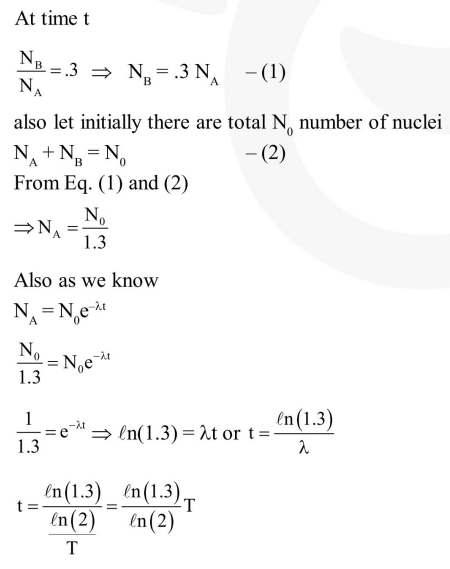# A radioactive nucleus A with a half life`
Question:

A radioactive nucleus $\mathrm{A}$ with a half life $\mathrm{T}$, decays into a nucleus $\mathrm{B}$. At $\mathrm{t}=0$, there is no nucleus B. At sometime $t$, the ratio of the number of $B$ to that of $A$ is $0.3$. Then, $t$ is given by :

1. $\mathrm{t}=\mathrm{T} \log (1.3)$

2. $t=\frac{T}{\log (1.3)}$

3. $t=\frac{T}{2} \frac{\log 2}{\log 1.3}$

4. $t=T \frac{\log 1.3}{\log 2}$

Correct Option: , 4

Solution: